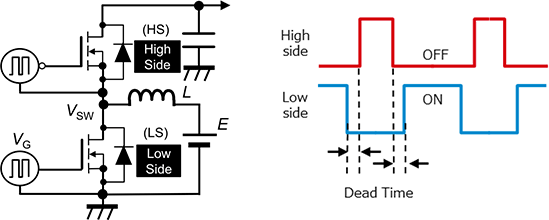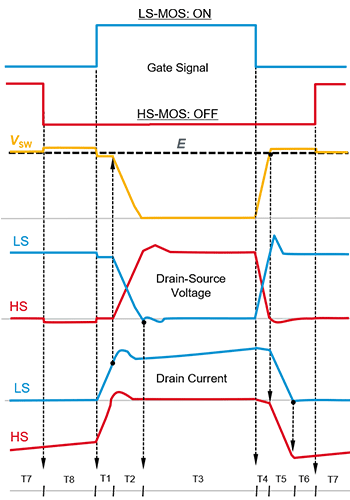Technical Information Site of Power Supply Design

2020.08.05 SiC Power Device

# SiC MOSFET Bridge Configuration

SiC MOSFETs: Behavior of Gate-Source Voltage in a Bridge Configuration

As part of our examination of " SiC MOSFET: Behavior of Gate-Source Voltage in Bridge Configuration", in this article we explain the bridge configuration that is assumed and its operation.

SiC MOSFET Bridge Configuration

The circuit below is the simplest synchronous boost circuit that uses MOSFETs in a bridge configuration. The high-side (HS) and low-side (LS) SiC MOSFETs used in this circuit are turned on in alternation, and in order to prevent the simultaneous turn-on of both the HS and LS devices, a dead time is set, during which both the HS and the LS MOSFETs are turned off. The waveforms on the lower right indicate the timing of the gate signals (VG).The approximate waveform shapes of the drain-source voltage (VDS) and the drain current (ID) of the HS and LS MOSFETs in this circuit are shown below. These waveforms assume a so-called hard-switching state, in which the current of the inductor L produces continuous operation.

The horizontal axis represents time; the time periods Tk (k=1 to 8) are defined as follows.

• T1： Interval in which LS is on and the MOSFET current is changing
• T2： Interval in which LS is on and the MOSFET voltage is changing
• T3： Interval in which LS is on
• T4： Interval in which LS is off and the MOSFET voltage is changing
• T5： Interval in which LS is off and the MOSFET current is changing
• T4 to T6： Dead time intervals preceding turn-on of HS
• T7： Interval in which HS is turned on (synchronous rectification interval)
• T8： Dead time interval from turn-off of HS to turn-on of LSThe discussion beginning in the next article will assume the above, and so the operation of this bridge circuit, and the voltage and current waveforms, should be thoroughly grasped.

#### Key Points:

・In examining" SiC MOSFET: Behavior of Gate-Source Voltage in Bridge Configuration", the simplest synchronous boost circuit, using MOSFETs in a bridge configuration, is used as an example.

・The configuration and operation of the example circuit, and the voltage and current waveforms, should be understood.